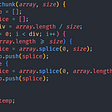# Letter Combinations of a Phone Number

In the ancient times, when there were no smartphones, the mobile phones used to have a keypad with digits ranging from 0–9 and some special characters like *, # and so on. Digits also had some alphabets along with them to give a messaging facility. Like here, if we want to type a, b, c we would need to press digit 2, one time, two times and three times respectively. So, we can type our whole message using the digits 2–9 as they cover whole range of character set i.e. from a-z.

## Problem Statement:

There is problem which is frequently asked in coding interviews that we are given a string containing digits from `2-9` inclusive, return all possible letter combinations that the number could represent.

For example:
digits = “23”

Let’s see how we can solve this problem.

## Approach

First of all, it is evident that all the digits ranging from 2–9 inclusive, can represent a set of alphabets that they can represent. Some digits like 2,3,4,5,6, and 8 can represent three alphabets while 7 and 9 can both represent 4 alphabets each. So, we first need to store that what all alphabets can be represented by a single digit.

## Character array

For that, I am using a character array of size 10. It will store the starting alphabet that our current digit can represent.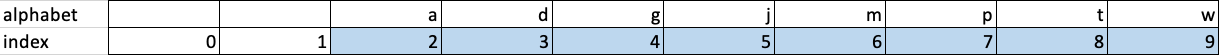Here, index of alphabet array represents the digit of keypad and value of alphabet represent starting alphabet for a particular digit
`private static char[] alphabet = new char;char c = 'a'; //starting characterfor(int i=2; i<10; i++){    alphabet[i]=c;    c = (i==7||i==9)?(char)(c+4):(char)(c+3);}`

Now, let’s go through some examples to have a better understanding.

Example 1: digits = “”
In this case, digits string is null, so we can’t make any letter combinations with zero digits, therefore return empty list

Example 2: digits = “2”
In this case, digits string has only one digit, so we can select only letters allowed from digit 2. As digit 2 can represent three alphabets, therefore the possible combinations will be “a”, “b”, and “c”, selecting one at a time.
Similarly, if digits = “7”, then, as digit 7 can represent four alphabets, therefore the possible combinations will be “p”, “q”, “r”, and “s”, selecting one at a time.

Example 3: digits = “23”
In this case, digits string has two digits in it, i.e. 2 and 3. Both 2 and 3 can represent 3 alphabets.
If we select, first alphabet corresponding to digit 2, i.e. ‘a’, then we can move to the next digit 3 and check out it’s all the possible representations.

So, fixing 2 = ‘a’, our tree would look like this: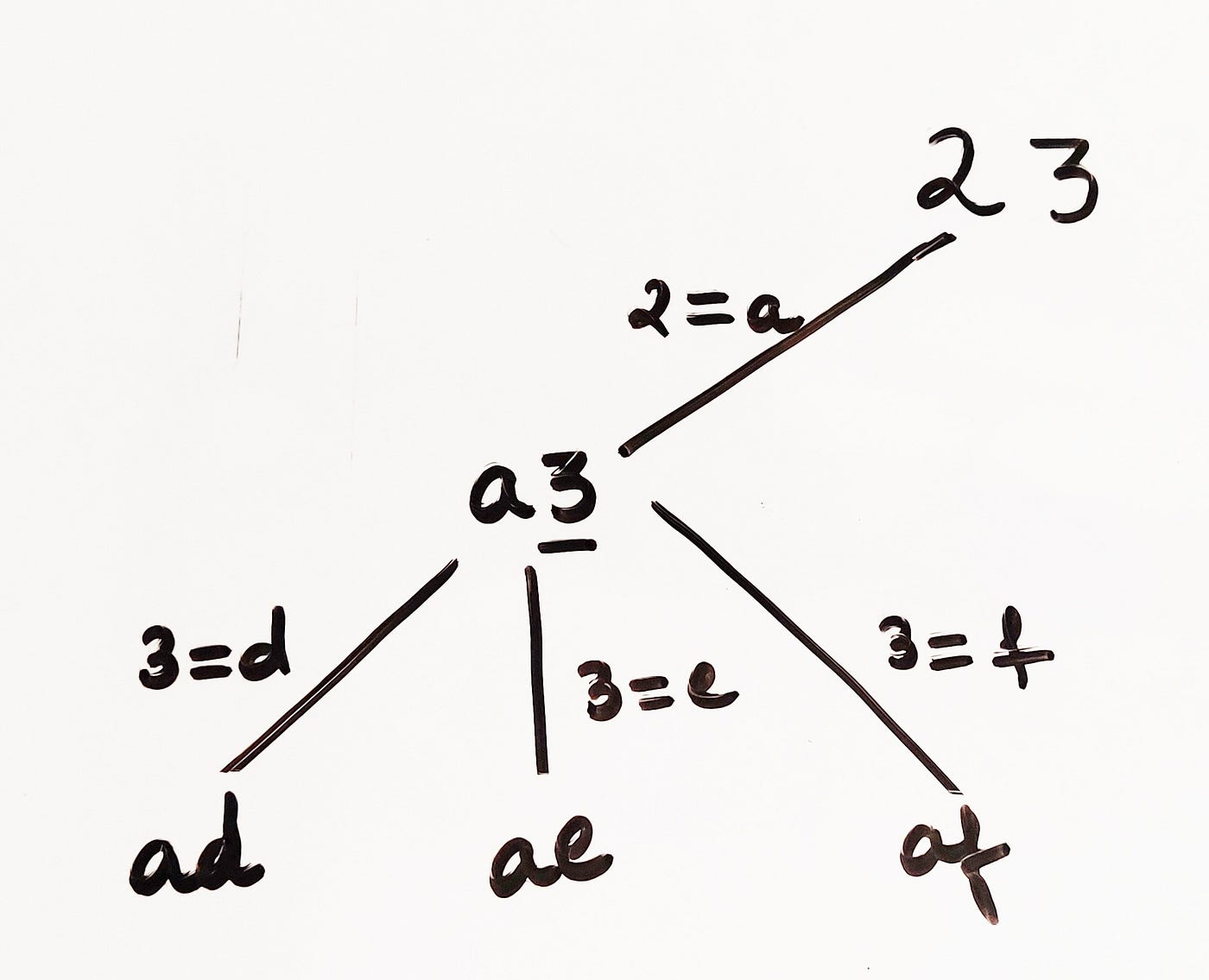Fixing 2 as ‘a’, we have three options for 3 i.e. (d,e and f)

Fixing 2 as ‘a’, we have three options for 3 i.e. (d,e and f). Therefore, (3+3+3) combinations are possible, i.e. 9 combinations, after fixing 2 as either a, b or c one at a time. Combinations: [ad, ae, af, bd, be, bf, cd, ce, cf]

Example 4: digits = “27”
In this case, digits string has two digits in it, i.e. 2 and 3. 2 can represent 3 alphabets whereas 7 can represent 4 alphabets.
If we select, first alphabet corresponding to digit 2, i.e. ‘a’, then we can move to the next digit 7 and check out it’s all the possible representations = [ap, aq, ar, as]. Same way as above, the total possibilities will be (4+4+4) = 12.

There is one observation that when we have two digits each with 3 possible character representation (e.g. 23) then no. of possible combinations = 3². When there is one digit representing 3 alphabets and one digit representing 4 alphabets, then no. of possible combinations = 3*4

Let’s take an example of three digits to generalize the no. of possible combinations.

Therefore, generalized formula for no. of combinations of letters possible for a digits string = 3^n * 4^m where, n=no. of digits that represents exactly 3 alphabets and m=no. of digits that represents exactly 4 alphabets.

For above example of 273 = no. of combinations = 3² * 4¹ = 36.

## Code analysis

The above problem can be solved using recursion and backtracking. We start with an empty StringBuilder in the beginning. Each digits in the digits string have possible representations of alphabets that it can represent.

Initialize start=0, representing the current position of digits string and list will be empty

`private void recursion(int start, String digits, StringBuilder sb, List<String> list){        //if we have used all digits of digits string, all the current letter combination in the list and return        if(start==digits.length()){            list.add(new String(sb.toString()));            return;        }                //compute the bound for current digit, i.e. how many alphabets current digit can represent, if current digit is 7 or 9, then it can represent 4 alphabets and the remaining digits can represent 3 alphabets        int bound = digits.charAt(start)-'0'==7 || digits.charAt(start)-'0'==9 ? 4: 3;                //starting from first alphabet for current digit to the last alphabet it can represent        for(int j=0; j<bound; j++){            //add the current alphabet to the string builder            sb.append((char)(alphabet[digits.charAt(start)-'0']+j));            //recurse for the next digit of digits string            recursion(start+1, digits, sb, list);            //backtrack and delete the current alphabet from the string builder            sb.deleteCharAt(sb.length()-1);        }    }`

Time Complexity = O(3^n * 4^m), as we have this many combinations explained above.
Space Complexity = O(3^n * 4^m), extra space to store these many combinations.

Guys I really hope that you find this article valuable! If still you have any queries, you can surely discuss them in the comment section below.

Thanks a lot for devoting your time to this blog.

Please share it among your colleagues and clap for showing appreciation! :)

--

--

--

## More from TRICK THE INTERVIEWER

Programming interview is the one where the interviewer will make all possible attempts to trick you. Trick the interviewer this time! :)

## cs371p Blog 10 Gavin Garcia## OPERATORS IN PYTHON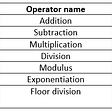## React Native Interview Questions — A Guide For The Founder (Part II)## Microservices Architecture & Containers — Part Two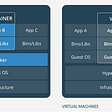## Bewakoof clone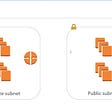## Connect Private Compute Instance using OCI Cloud Shell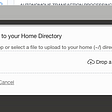## Task 02- Run GUI Application inside Docker Container In this blog , I am going to launch two GUI…## Jhanak Didwania

Software Engineer | Programmer

## Algorithms: Selection Sort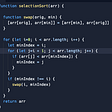## Array chunk algorithm